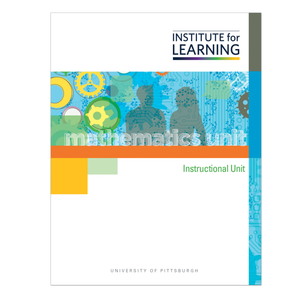# Grade 3: Multiplication Situations (CCSSM Version)

Regular price \$149.00 \$0.00 Unit price per

Concepts

• Equal Group Multiplication Situations
• Array/Area Model Multiplication Situations
• Measurement Multiplication Situations

Unit includes

• 14 high-level tasks with associated QuickStarts, Practices, Applications, and Quick Writes.
• 2 checkpoints and 4 support activities.
• 1 final performance-based assessment with scoring guide.

Students

• Explore and solve equal group multiplication situations.
• Understand and identify the meaning of the factors as one being the number of equal groups and the other as the group size.
• Use a variety of representations, including manipulatives, diagrams, finger counting, and the number line models, to make sense of multiplication and to arrive at the product.
• Explore, compare, and use various strategies for multiplying, including skip counting, the use of repeated addition, known multiplication equations, and related multiplication equations.
• Explore and solve array and area model situations.
• Understand that rows with items/square tiles or columns with items/square tiles represent a multiplication situation.
• Understand that an area, when rotated, still represents the same product or area.
• Explore and solve measurement multiplication situations.
• Recognize that iterations of measures can represent equal groups.